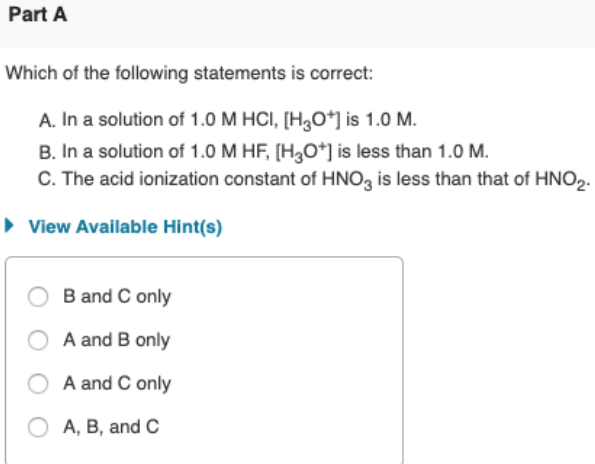# Which of the following statements is correct: A. In a solution of 1.0 M HCl, [H3O^+] is 1.0 M. B. In a solution of 1.0 M HF, [H3O^+] is less than 1.0 M. C. The acid ionization constant of HNO3 is less than that of HNO2. a) B and C only b) A and B only c) A and C only d) A, B and C